Home
Hostname: page-component-768dbb666b-x9ds4 Total loading time: 0.389 Render date: 2023-02-06T04:17:34.902Z Has data issue: true Feature Flags: { "useRatesEcommerce": false } hasContentIssue true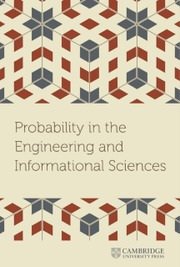Probability in the Engineering and Informational Sciences

# EXACT ASYMPTOTICS OF SAMPLE-MEAN-RELATED RARE-EVENT PROBABILITIES

Published online by Cambridge University Press:  16 January 2017

## Abstract

Relying only on the classical Bahadur–Rao approximation for large deviations (LDs) of univariate sample means, we derive strong LD approximations for probabilities involving two sets of sample means. The main result concerns the exact asymptotics (as n→∞) of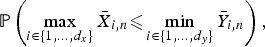$${\open P}\left({\max_{i\in\{1,\ldots,d_x\}}\bar X_{i,n} \les \min_{i\in\{1,\ldots,d_y\}}\bar Y_{i,n}}\right),$$
with the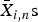${\bar X}_{i,n}{\rm s}$ (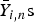${\bar Y}_{i,n}{\rm s}$, respectively) denoting dx (dy) independent copies of sample means associated with the random variable X (Y). Assuming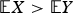${\open E}X \gt {\open E}Y$ , this is a rare event probability that vanishes essentially exponentially, but with an additional polynomial term. We also point out how the probability of interest can be estimated using importance sampling in a logarithmically efficient way. To demonstrate the usefulness of the result, we show how it can be applied to compare the order statistics of the sample means of the two populations. This has various applications, for instance in queuing or packing problems.

Type
Research Article
Information

## Access options

Get access to the full version of this content by using one of the access options below. (Log in options will check for institutional or personal access. Content may require purchase if you do not have access.)

## References

1. Andriani, C. & Baldi, P. (1997). Sharp estimates of deviations of the sample mean in many dimensions. Annales de l'Institut Henri Poincare (B) Probability and Statistics 33(3): 371385.CrossRefGoogle Scholar
2. Asmussen, S. & Glynn, P.W. (2007). Stochastic simulation: algorithms and analysis. Stochastic Modelling and Applied Probability. New York: Springer.Google Scholar
3. Bahadur, R.R. & Rao, R.R. (1960). On deviations of the sample mean. The Annals of Mathematical Statistics 31(4): 10151027.CrossRefGoogle Scholar
4. Chaganty, N.R. (1997). Large deviations for joint distributions and statistical applications. Sankhyā: The Indian Journal of Statistics, Series A 59: 147166.Google Scholar
5. Chaganty, N.R. & Sethuraman, J. (1993). Strong large deviation and local limit theorems. The Annals of Probability 21(3): 16711690.CrossRefGoogle Scholar
6. Chaganty, N.R. & Sethuraman, J. (1996). Multidimensional strong large deviation theorems. Journal of Statistical Planning and Inference 55(3): 265280.CrossRefGoogle Scholar
7. Dembo, A. & Zeitouni, O. (1998). Large deviations techniques and applications, 2nd ed. New York: Springer-Verlag.CrossRefGoogle Scholar
8. Glynn, P. & Juneja, S. (2004). A large deviations perspective on ordinal optimization. In Proceedings of the 2004 Winter Simulation Conference. Vol. 1, IEEE.Google Scholar
9. Glynn, P. & Juneja, S. (2015). Ordinal optimization-empirical large deviations rate estimators, and stochastic multi-armed bandits. arXiv preprint arXiv:1507.04564.Google Scholar
10. Höglund, T. (1979). A unified formulation of the central limit theorem for small and large deviations from the mean. Zeitschrift für Wahrscheinlichkeitstheorie und verwandte Gebiete 49(1): 105117.CrossRefGoogle Scholar
11. Iltis, M. (1995). Sharp asymptotics of large deviations in ℝ d . Journal of Theoretical Probability 8(3): 501522.CrossRefGoogle Scholar
12. Mandjes, M. (2007). Large deviations for Gaussian queues. Chichester, UK: John Wiley & Sons, Ltd,.CrossRefGoogle Scholar
13. Petrov, V.V. (1975). Sums of independent random variables. New York: Springer-Verlag.CrossRefGoogle Scholar
14. Sadowsky, J.S. & Bucklew, J.A. (1990). On large deviations theory and asymptotically efficient Monte Carlo estimation. IEEE Transactions on Information Theory 36(3): 579588.CrossRefGoogle Scholar
2
Cited by

# Save article to Kindle

Note you can select to save to either the @free.kindle.com or @kindle.com variations. ‘@free.kindle.com’ emails are free but can only be saved to your device when it is connected to wi-fi. ‘@kindle.com’ emails can be delivered even when you are not connected to wi-fi, but note that service fees apply.

Find out more about the Kindle Personal Document Service.

EXACT ASYMPTOTICS OF SAMPLE-MEAN-RELATED RARE-EVENT PROBABILITIES
Available formats
×

# Save article to Dropbox

To save this article to your Dropbox account, please select one or more formats and confirm that you agree to abide by our usage policies. If this is the first time you used this feature, you will be asked to authorise Cambridge Core to connect with your Dropbox account. Find out more about saving content to Dropbox.

EXACT ASYMPTOTICS OF SAMPLE-MEAN-RELATED RARE-EVENT PROBABILITIES
Available formats
×

# Save article to Google Drive

To save this article to your Google Drive account, please select one or more formats and confirm that you agree to abide by our usage policies. If this is the first time you used this feature, you will be asked to authorise Cambridge Core to connect with your Google Drive account. Find out more about saving content to Google Drive.

EXACT ASYMPTOTICS OF SAMPLE-MEAN-RELATED RARE-EVENT PROBABILITIES
Available formats
×
×